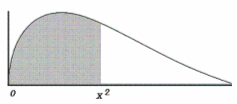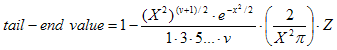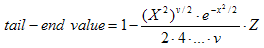Chi Square Calculator - Online

Below you can find a Chi-square calculator. In probability and statistics, the chi-square distribution with v degrees of freedom is the distribution of a sum of v2 independent standard normal random variables. It’s one of the most
used probability distributions in inferential statistics.

This spreadsheet calculates the tail-end value for points on a chi-square (X2) distribution curve. You must enter the X2 value and the degrees of freedom.Chi-square distribution curve

The unshaded area represents the tail-end value of X2. The shaded area represents the percentile.

The X2 distribution function is calculated using the following formulas:

with v odd,with v even,where

v = degrees of freedomExample: let's calculate percentiles and tail-end values

A X2 statistic for a given study was computed to be 3.2 with two degrees of freedom. What are the tail-end and percentile values?

Enter degrees of freedom = 2
Enter chi-square (X2) = 3.2

and get these results:

Tail end value = 0.2019
Percentile =      0.7981

 Please, enable JavaScript codes on this page, othewise the calculator won't work at all.Chi-square Distribution Enter degrees of freedom: Enter Chi-square value: Tail-end value: Percentile:

From 'Chi square Calculator' to home

From 'Chi square Calculator' to Free Online Calculators

 Top Chi-square code in Matlab Probability and Stats